# Pratima Nayak

•### Opposite angles of cyclic quadrilateral

Activity

Pratima Nayak

•### Move the point and find the coordinates

Activity

Pratima Nayak

•### quiz exponent rule level-1

Activity

Pratima Nayak

•### quiz percentage conversion

Activity

Pratima Nayak

•### quiz exponent rule level-3

Activity

Pratima Nayak

•### Integer games for challengers

Activity

Pratima Nayak

•### CBSE SECONDARY MATHEMATICS

Book

Pratima Nayak

•### Know the parts of a circle

Activity

Pratima Nayak

•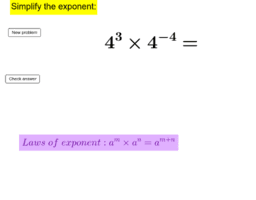### Laws of exponent worksheet-2

Activity

Pratima Nayak

•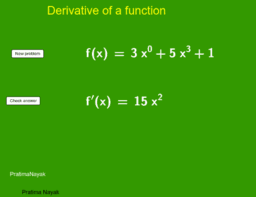### Practice material for derivative of a function

Activity

Pratima Nayak

•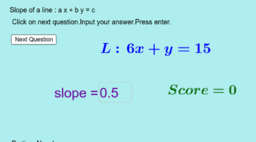### Worksheet Slope of a line.

Activity

Pratima Nayak

•### Multiplication Table worksheet

Activity

Pratima Nayak

•### Click on new problem and learn LCM

Activity

Pratima Nayak

•### Click on the new and learn GCD

Activity

Pratima Nayak

•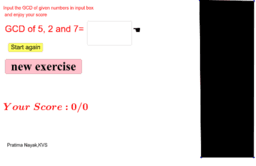### GCD of three numbers

Activity

Pratima Nayak

•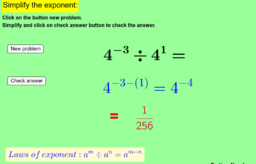### Laws of exponent Practice sheet-1

Activity

Pratima Nayak

•### Prime before and after

Activity

Pratima Nayak

•### Prime factors of a number practice material

Activity

Pratima Nayak

•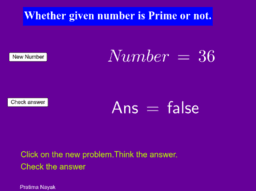### Prime Numbers practice material

Activity

Pratima Nayak

•### What percent of a is b?

Activity

Pratima Nayak

•### word problems related todigits classVIIICBSE

Activity

Pratima Nayak

•### word problem- 2 related to digits classVIIICBSE

Activity

Pratima Nayak

•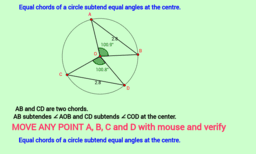### Equal chords subtend equal angles at the center.

Activity

Pratima Nayak

•### wordproblemsrelatedtodigit&numbers

Activity

Pratima Nayak